Next: 2.4 Programming languages
Up: 2.3 Numerical analysis
Previous: 2.3.1 Numerical Recipes
[ID index][Keyword index]

### 2.3.2 Floating point representation

As with numerical analysis, the intricacies of how floating-point numbers are represented, and the quirks of their representation on different platforms, are a maelstrom into which you can fall, dazed, confused and unproductive. However (and again as with numerical analysis), if your codes become elaborate enough, then you are going to have to take the plunge.

Even if you have no plans to venture into the deep, there is a minimum awareness of the problems which can help you write more robust code.

What follows here is rather detailed, but it does, I believe, represent the majority of what you might need to know about floating point representation; there is a good alternative introduction in Numerical Recipes [nr], and an excellent and detailed introduction in chapter 2 of Sun's Numerical Computation Guide [sunncg] (appendix E of the same book claims to be What every computer scientist should know about floating point arithmetic', and is an edited reprint of [goldberg91]. It makes interesting reading, but it's probably more than many natural scientists need to know). My account here is not intended to supplant either of these sources. Below, I'll talk exclusively of IEEE base-2 floating point numbers, as defined in IEEE standard IEEE-754; there are other standards, but they're now of historical interest only, as just about all modern machines other than VAXes and older Crays use IEEE. Most of what I say concerning accuracy will be about single precision; exactly the same issues arise with double precision, but you can sweep them under the carpet for longer.

#### 2.3.2.1 Endianness of floating-point numbers

The IEEE standard leaves it open to chip manufacturers to decide the order in memory of the four or eight bytes of a floating point number (the same is true of integers, though they're not part of the IEEE spec). This is denoted by the endian-ness (or bytesex') of the machine. Alpha and Intel chips are little-endian; Sparcs, the Motorola 68k family used in Macs, and Motorola PowerPC chips, are big-endian (there's also a PGP-endian' ordering, but that really isn't likely to trouble anyone any more). This generally does not matter if you stick to a single platform (other than in the context of programs such as those described in Appendix A.1 and Appendix A.2), but it makes a difference if you want to share raw data files (see Section 2.5.2.3) between architectures.

#### 2.3.2.2 Accuracy

The central insight is that you cannot represent an infinite number of reals in a finite number of bits without approximation. It is a consequence of this that the result of any calculation will lose precision when it is represented as a floating-point bit pattern, and that the extent and importance of this loss of precision depends on the operands. Both of these seem obvious by themselves, but the consequences can be sufficiently non-obvious to trip you up.

A non-special IEEE floating-point single-precision longword represents a numberwhere(the sign),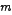(the mantissa or significand) and(the exponent) are base-2 numbers represented in 1, 8 and 23 bits respectively, and; the offset 127 is called the bias. These are encoded into the 32 bits of the longword as shown in table Table 1.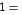0 0 1 1 1 1 1 1 1 0 0 0 0 0 0 0 0 0 0 0 0 0 0 0 0 0 0 0 0 0 0 00 1 0 0 0 0 0 0 0 0 0 0 0 0 0 0 0 0 0 0 0 0 0 0 0 0 0 0 0 0 0 0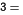0 1 0 0 0 0 0 0 0 1 0 0 0 0 0 0 0 0 0 0 0 0 0 0 0 0 0 0 0 0 0 0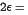0 0 1 1 0 1 0 0 0 0 0 0 0 0 0 0 0 0 0 0 0 0 0 0 0 0 0 0 0 0 0 0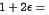0 0 1 1 1 1 1 1 1 0 0 0 0 0 0 0 0 0 0 0 0 0 0 0 0 0 0 0 0 0 0 1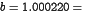0 0 1 1 1 1 1 1 1 0 0 0 0 0 0 0 0 0 0 0 0 1 1 1 0 0 1 1 0 0 1 1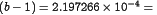0 0 1 1 1 0 0 1 0 1 1 0 0 1 1 0 0 1 1 0 0 1 1 0 0 1 1 0 0 1 1 0

Table 1

IEEE floating-point representations of numbers. This table is adapted from table 2--3 in [sunncg] and figure 1.3.1 in [nr], which have fuller discussions of the issues here. For discussion ofandsee the text.

Firstly, it is important to distinguish between the range and the accuracy of floating-point numbers. The smallest and largest positive numbers which can be represented by normal IEEE single-precision numbers are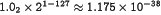and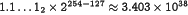, but this is very different from the accuracy to which these numbers are represented. These extreme values differ from the next representable ones up and down byand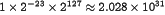respectively, so that any number which differs from them by less than that amount is unrepresentable. This accuracy limitation is expressed by the machine epsilon,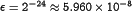(for IEEE single-precision), which is the maximum relative error incurred when a real number is represented in floating-point. It is a consequence of this thatis the smallest number such that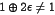, where the operator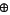represents addition of floating-point numbers.[Note 1]

Another way of thinking about this is as follows. When two floating-point numbers are added, the exponent of the smaller one must be increased to match that of the larger, and the mantissa (or significand),, must be shifted rightwards to compensate. If the two numbers differ greatly in magnitude, then the smaller will lose significance in this operation, and if it is less than a factorof the larger one, the shift will push the number right off the end of the mantissa, and contribute nothing to the sum. You can easily confirm that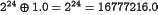.

The immediate practical consequence of this is that if, in the bowels of some calculation, you are adding up many small numbers (doing an inner product for very large vectors, for example), then the final total may be grossly inaccurate if you've fallen into this trap. Similarly, if you are subtracting numbers which are almost equal, perhaps in the course of debiasing or removing some DC offset, the subtraction may allow all or most of the leading accurate digits to cancel, leaving the result to be determined by digits possibly heavily contaminated by roundoff. [RW]

Consider the simple calculation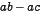, with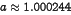,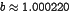and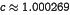. Here, the rounding in the two multiplications conspires to give a difference which has a huge relative error of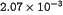. We can, however, address the problem by rewriting the calculation in ways which are equivalent for real arithmetic, but inequivalent for machine arithmetic. If we do the subtraction before the multiplication, and calculate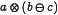, then we retain a little precision in the subtraction and end up with a relative error of. Finally, if we instead calculate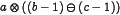, then we do the subtraction with a full 23 bits of precision (compare Table 1), and lose as little as possible of this in the final multiplication, and end up with a relative error of only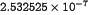. This is clearly a rather synthetic example, but it is not at all unrealistic, as it is equally clearly closely related to the common problem of calculating the discriminant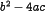of the quadratic formula, when the quadratic is nearly degenerate.

These problems are demonstrated in the short program fpdemo.c in Appendix A.3. Note that the numbers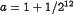,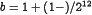and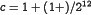are (a) specifically chosen to demonstrate the effect, and (b) are calculated within the program, rather than being initialised from the decimal representations 1.000244, and so on. If the latter were not true, then the improvement in relative error would disappear, since all precision would have been lost in this initialisation. If you wish to explore the representation of floating-point numbers on your machine, you can do so using the example program fpp.c in Appendix A.2.

These problems are unfortunately both easy to run into, and hard to notice if you're not in the habit of looking for them whilst writing your code. A brute-force way around them is to do sensitive parts of your calculation in double precision. If you are doing the calculation in double precision and still running into the problem, then you will have to rearrange the calculation somehow, to contain the loss of precision.[Note 2] Note, however, that a compiler's optimizer can frustrate your efforts here, if you've given it a sufficiently high optimization level that it starts reorganising your code: in general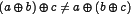, and, but a high optimization level may instruct the compiler to ignore this fact, which can be disastrous for the accuracy of your code. Moral: make sure you know what the various optimization levels actually do before you invoke them.

As alluded to above, it is generally possible to sweep accuracy problems under the carpet by using double-precision floats. These occupy eight bytes of storage rather than four, and the analogue of Eqn.(1) is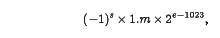where,andare respectively 1, 52 and 11 bits long, and the bias is 1023. Thus the smallest and largest normal positive double-precision IEEE numbers are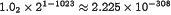and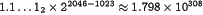, and the machine epsilon for double precision is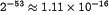.

On some platforms such as Solaris and Compaq, with the manufacturer's compilers, it is possible to use quadruple precision. You should not use double or quadruple precision automatically, however, for a variety of practical and principled reasons.

Firstly, if your program already suffers from rather prodigal use of memory (ie, has very large arrays), then the doubling in the size of real arrays will only make the problem worse and, by probably increasing the amount of swapping, might make your program significantly slower.

Secondly, although there are some issues to do with the relative speed of single- and double-precision calculations, these are probably not significant enough to be worth worrying about in all but the most long-running codes, and in any case would generally be swept up by the compiler (I'd welcome any correction or amplification on this point).[Note 3]

Thirdly, if your results change if you switch to double-precision, then there is an accuracy problem in your code, which would probably bear some investigation. If there is some part of your code which genuinely requires the extra precision -- say because you have to add numbers of hugely different scale in accumulating a large inner product -- then there is not much you can do about it, and that part of your code, at least, must have the extra precision. Failing such an explanation, however, you can regard such behaviour as a miner's canary, indicating some potential problem with your code, which you might benefit from investigating further.

Fourthly, remember that some algorithms are designed to work with single precision, and will no longer work properly if you search-and-replace double for float or real*8 for real*4. As just one example, one of the Numerical Recipes algorithms (svdcmp) includes a convergence test of, essentially, if (val+err==val). Whatever the merits or otherwise of this as a test, it is a cheap way of establishing whether err has reached machine precision, which will not work in the expected way if val and err are double precision.

For further details on floating point representation, see, for example [hauser96], and informal but very useful discussions (both available on the web) by William Kahan [kahan96] and Jim Demmel [demmel]. The former is one of the authors of the IEEE-754 standard for floating-point numbers.

#### 2.3.2.3 Other floating-point topics

As well as defining the format in which normal floating-point numbers are stored, the IEEE-754 standard includes the definitions for several other (classes of) special values.

When the exponent(of a single-precision number) is zero, the longword is taken to represent the number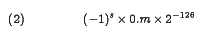(compare Eqn.(1)). Unlike the usual floating-point numbers, which have an implied leading 1 in the significand and 23 bits of precision, and which are referred to as normalised', these have an implied leading 0 and less than 23 bits of precision. These are denormal' or subnormal' numbers, and are the result of an underflow, such as dividing the smallest normalised number by two. This behaviour is known as gradual underflow', and allows the precision in a calculation to degrade gracefully when it underflows. It is distinct from Store-0 underflow' common before the IEEE standard, in which any expression which underflowed was replaced by zero. This was, and remains, one of the more contentious parts of the IEEE standard. Be warned that older Crays, which use their own floating-point format, have a Store-0 underflow policy, and that the Alpha chip, although it generally implements IEEE floats, has a Store-0 underflow as its default mode, and will neither produce, nor accept as input, denormalised numbers.

If the significand as well as the exponent is zero, the longword represents the number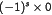. Note that the IEEE zero is signed, which allows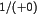and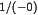to be positive and negative infinity; this can be important when doing calculations near branch points.

If the exponent is 255 and the significand is zero, the longword represents the value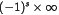. That is, infinity has a special value, distinct from the largest possible normalised number. Positive infinity is generated by, for example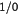, or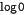, where infinity is the mathematically correct, but otherwise unrepresentable, value of a calculation.

If the exponent is 255 and the significand is non-zero, the longword is Not a Number, usually represented by the string NaN'. A NaN is the result of an operation on invalid operands, such as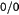or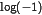. NaNs have the properties that any operation which has a NaN as an operand has a NaN as its result; and any comparison on a NaN, such as < or ==, evaluates to False, including NaN==NaN. The only exception is that, when x is a NaN, then x != x is true. Why would you want to use such a peculiar number? Generally you don't, and its appearance in a calculation is an error, which is why processors can be set to dump core when a NaN or an Infinity is produced. However, it can be useful to turn off this behaviour if it is not off by default, and rather than elaborately avoid producing a NaN at each stage, make a check once at the end of a calculation, possibly invoking an alternative (possibly more expensive or special-cased) algorithm if any NaNs are found.

Different compilers handle exceptional values in different ways; see Table 2. Of the three Starlink platforms, only the Alpha traps on exceptional values by default.

Compilertraps enabled by defaultseesuppress with
Sun cc & f77none-ftrap
Alpha ccoverflow, divide-by-zero, invalid operation-fptm, -ieee-ieee
Alpha f77overflow, divide-by-zero, invalid operation-fpe-fpe1
gccnone-mfp-trap-mode (Alpha-gcc)

Table 2

Summary of compiler IEEE traps

We have only scratched the surface of a rather intricate topic here. Both Suns and Alphas have extensive f77 and cc` man-pages, which hint at the broad range of floating-point options available when compiling and linking your code. See Section 2.5.2.4 for discussion of the efficiency tradeoffs when using IEEE exceptional values.Next: 2.4 Programming languages
Up: 2.3 Numerical analysis
Previous: 2.3.1 Numerical Recipes
[ID index][Keyword index]
Theory and Modelling Resources Cookbook